# Time Series Forecasting With TensorFlow and InfluxDB

This article was originally published in The New Stack and is reposted here with permission.

You may be familiar with live examples of machine learning (ML) and deep learning (DL) technologies, like face recognition, optical character recognition OCR, the Python language translator, and natural language search (NLS).

But now, DL and ML are working toward predicting things like the stock market, weather and credit fraud with astounding accuracy. As you may have noticed, these categories are all time-dependent and fall within the category of time-series data.

Time-series data refers to a set of values that change over time or can be expressed in terms of time. With time-series data, you’re always dealing with large amounts of data that needs to be stored over long periods. Storing time-series data in a relational database can be difficult at scale due to scalability issues. This is where time-series databases (TSDBs) come in.

TSDBs are designed specifically for storing time-series data. InfluxDB is a widely used TSDB that tracks measurements and events over time and stores them based on aggregated time.

However, after you store your data with InfluxDB, your work isn’t done. Data is only useful when you can analyze and use it to improve your business. For instance, you can use time-series data to forecast future weather patterns in a process known as time-series forecasting.

In this article, you’ll learn how data from InfluxDB can be used to train a model in TensorFlow and make predictions.

## What Is InfluxDB?

InfluxDB’s data model is specifically intended for time-series data and, therefore, doesn’t have any limitations like replacing older values with newer ones or higher-access times. It uses tags and leaves fields unindexed, which automatically ensures you’ll have good database performance. InfluxDB is versatile and supports a wide range of data types and lets the user create additional fields and tags as needed.

InfluxDB can be used in a multitude of time-sensitive scenarios, including the following:

• Stock market: Relational databases store data in batches many entries at a time, whereas stock prices need to be stored one row at a time because you don’t have the following day’s price. If you use InfluxDB to store stock market data over time, it provides you with both speed and efficiency. Once this data is stored, you can apply different time-series algorithms, like Autoregressive Integrated Moving Average (ARIMA) or a neural network approach to forecast stock price values for upcoming days. (Please note: This is not 100% accurate, but it gives you an idea of where the market may go.)
• Health monitoring: Devices like smartwatches and phones are used to track your health. In the backend of these devices, ML/DL algorithms are applied to the data to make necessary predictions. If any anomaly is found in the health of the user, their doctor is informed about the changes.
• Weather data: Multiple sensors can store weather data for a specific period. If stored in InfluxDB, the data can be used with different algorithms to help make forecasts. However, not all the data that is stored in the database can be used for making predictions since it would be too exhaustive. In this case, a specific time frame is selected, such as two months’ worth of data, then different statistical methods, like ARIMA, ARIMAX or SARIMAX, are used to forecast the weather.

## Time series forecasting with TensorFlow and InfluxDB

As mentioned previously, you’re going to connect to InfluxDB and install an InfluxDB Python library. Then you’ll use a dataset and build a model from the data to make some predictions. The first thing you need to do is set up your InfluxDB account.

This tutorial assumes you’re using a macOS interface, but the instructions are similar if you’re working with Windows or Unix as well.

## Setting up InfluxDB

To install InfluxDB in your macOS, you can use Homebrew:

``````\$ brew update
\$ brew install influxdb influxdb-cli``````

Alternatively, you can manually download it from the Install InfluxDB page or sign up for a free InfluxDB Cloud account to get started with no local installation required.

Note: if you experience a Too many open files error, follow these steps to fix it.

Once installed, start InfluxDB with the following command in your terminal:

``influxd``

When you start InfluxDB for the first time, you need to feed it some required values or you won’t be able to use it. You can set it up using either command lines terminal or localhost GUI. To configure InfluxDB through a terminal, use the setup command:

``\$ influx setup``

For the initial setup, the following details will be required:

• Organization name: An organization name is required for database operations.
• Bucket name: You can have as many buckets as you want for an organization, but in the initial setup, you need to create at least one bucket.
• Retention period: This is the period in which your bucket will store data and then automatically delete it. If you select Never or leave it empty, it stores data for an infinite period.

If you want to configure the InfluxDB using GUI, you need to access localhost:8086localhost:8086. Once there, it will ask you for all the required details previously mentioned.

To set up InfluxDB on other platforms, you can refer to the Install InfluxDB page for more information.

Once the initial setup is done and your account has been created, you need to login to localhost:8086localhost:8086, and you should see the following: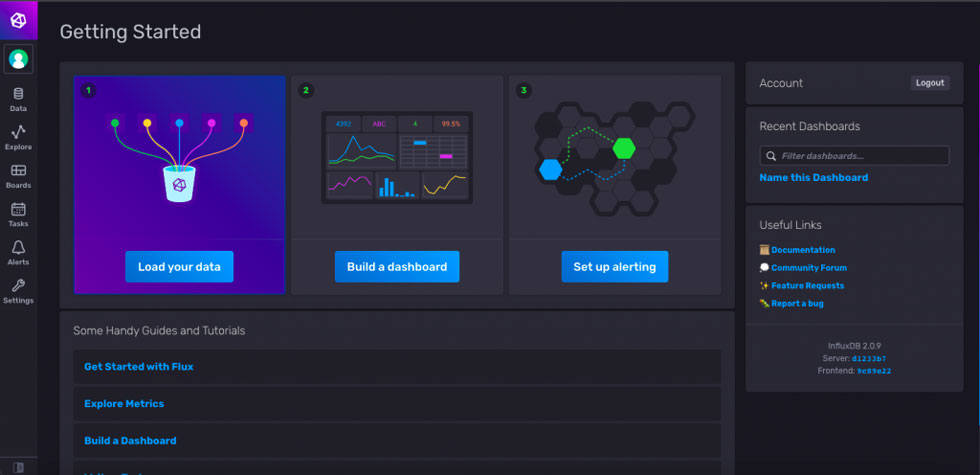In this tutorial, you only need to focus on the Data component once you’re connected to the database. However, you can explore all the options available on the dashboard if you wish to do so.

Now, click on the Data icon on the left-hand sidebar, and you’ll see a screen like this: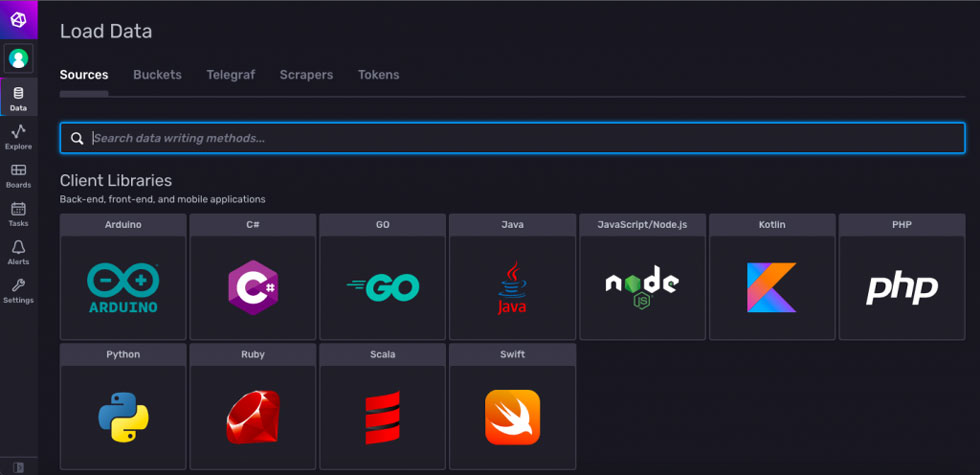In order to work with Python the TensorFlow library, you need to review the Buckets and Tokens section. Buckets are like database names similar to what you have in relational databases, and Tokens are unique keys that are only accessible for different database operations.

In this case, you’ve already created an initial bucket. To generate the unique token, navigate to the Tokens section and click on the Generate Tokens button. There are two different types of tokens that you can use: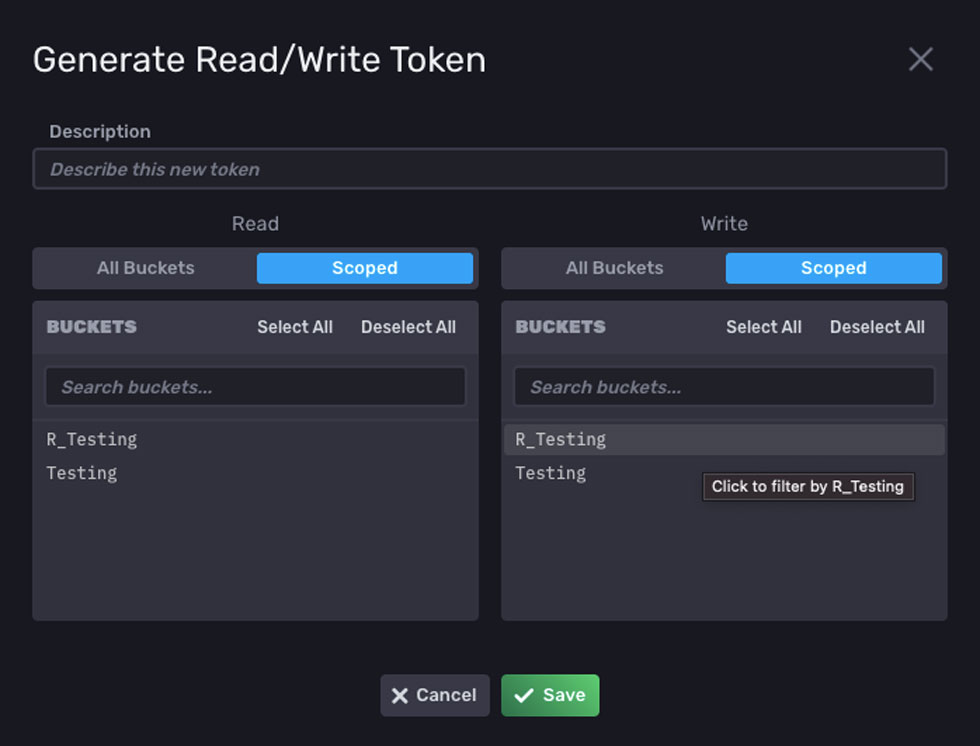1. All Access Token: This token gives you full control of the database. You can perform read, write, update and delete operations to any bucket present in InfluxDB. In this tutorial, you only need to use this token since it’s more flexible than read/write tokens: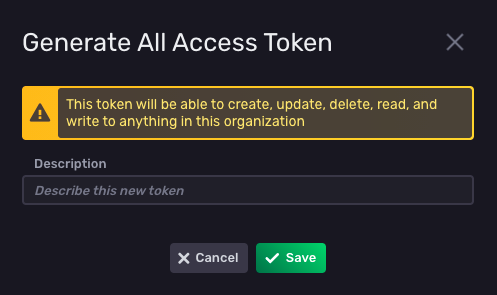Now that you have InfluxDB set up, it’s time to implement time-series forecasting with your data. Python 3.9 is used, along with Jupyter Notebook, for the development.

## Installing the InfluxDB Python Library

To install the InfluxDB Python library, you can use the Python package manager (pip) in your terminal or in Jupyter Notebook:

``````## install using terminal
\$ pip install influxdb-client

## install using jupyter notebook
! pip install influxdb-client``````

## Installing TensorFlow

TensorFlow is a very powerful library, and you can use it to implement any type of neural network, like an artificial neural network (ANN)], a convolutional neural network (CNN), or a recurrent neural network (RNN). To use it, you need to import different layers and models that you want to work with, compile them and then run them to get the trained model. To install TensorFlow using pip, run the following command:

``````## install using terminal
\$ pip install tensorflow

## install using jupyter-notebook
! pip install tensorflow
``````

## Exploring the dataset

In this tutorial, you’ll use a popular dataset that contains identified sunspots over a specific period. The code you will see in this tutorial is referenced from the article “Time Series Forecasting using TensorFlow and Deep Hybrid Learning.” You can download it from this GitHub repo.

1. Date: The date when the spots were recorded.
2. Monthly Mean Total Sunspot Number: The average number of sunspots recorded over time.

To read the dataset in Python and check the first few rows of data, use the following code:

(code)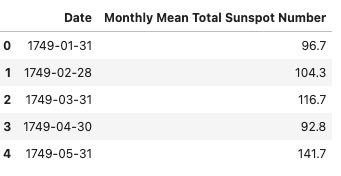### Connecting the dataset to InfluxDB

Before you can connect your data to InfluxDB, you need to make a connection to your bucket using the InfluxDB 2.0 Python client with the following code:

``````## import dependencies
from influxdb_client import InfluxDBClient, Point, WritePrecision
from influxdb_client.client.write_api import SYNCHRONOUS

## You can generate a Token from the "Tokens Tab" in the UI
token = "your-token"
org = "my-org"
bucket = "Testing"

## connect to influxdb
client = InfluxDBClient(url="http://localhost:8086", token=token, org = org)``````

The first two lines show the important imports for using InfluxDB. Then you define basic connection details, like the token, organization name, and bucket name where you want to store the data. Finally, you call the InfluxDBClient function to connect to InfluxDB. Here, the localhost URL is mentioned because you want to make a connection to a local InfluxDB client.

If you want to connect to the InfluxDB Cloud instance, you need to specify the respective URL. You can find different endpoints on the InfluxDB Cloud regions page.

## Inserting data

Now that you’ve connected to InfluxDB, you need to perform one more task before storing the data. InfluxDB assumes that the index in your dataset will be timestamp values; if they are not, it assumes the columns as different events and stores them at the current timestamp (current date and time). You can make the timestamp column as an index using the following code:

``````# convert Date column to datetime
data['Date'] = pd.to_datetime(data['Date'])

## create date as index
data.set_index(data['Date'], drop = True, inplace = True)
data.drop('Date', axis = 1, inplace = True)``````

Check out this Pandas and InfluxDB tutorial if you are interested in learning more about using Pandas with InfluxDB.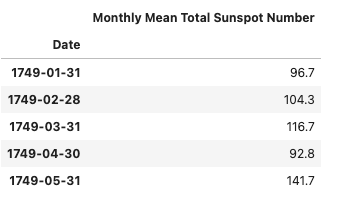To insert the data in InfluxDB using Python, you need to create a Write API object:

``````## create object of write API
write_api = client.write_api(write_options=SYNCHRONOUS)``````

Here, SYNCHRONOUS specifies that you will be storing multiple rows of data at a time. Now, you just need to pass your data frame to your write_api object:

``````## write data to influxdb
response = write_api.write(bucket, record = data, data_frame_measurement_name='sunspot',
data_frame_tag_columns=['sunspot'])``````

In the previous code, data_frame_tag_columns is used to store the columns’ metadata information, and data_frame_measurement_name is similar to the table name in the relational database. If the response from the write API is None, then your data is stored successfully; otherwise, you’ll receive an error message.

Note: Depending on your system capabilities, you may encounter a Too many open files error. In this case, you need to store some fixed number of rows at a time instead of passing the whole dataset at once:

``````i_lower = 0
## iterate over 25 rows at once and store it in influxdb
for i in range(0, len(data), 25):
response = write_api.write(bucket, record = data[i_lower:i], data_frame_measurement_name='sunspot',
data_frame_tag_columns=['sunspot'])

i_lower = i
print('%d rows inserted'%(i))``````

Now that you’ve stored your data in the InfluxDB database, you can read that data. To query the data from InfluxDB, you need to create a `read API` object. Flux is the easiest way to query your data from the database. You just need to specify the period you want to query:

``````## query data
query_api = client.query_api()
tables = query_api.query('from(bucket:"Testing") |> range(start: -275y)')``````

Here, the period is defined as `-275y` because you want to select all the entries for the past 275 years.

Once you’ve queried the data, you can iterate over each row to create a dataframe from that data:

``````## iterate over queried data
time, sunspot = [], []
for table in tables:
for row in table.records:
time.append(row.values.get('_time'))
sunspot.append(row.values.get('_value'))

## create dataframe
data = pd.DataFrame({'Date':time, 'Monthly Mean Total Sunspot Number': sunspot})
``````

## Inspecting and cleaning the dataset

Now that you have your data, you need to retrieve and clean the data from the Date column:

``````## convert datetime to only date
data['Date'] = data['Date'].dt.date``````

To visualize the sunspots data against the Date column, you can use the following code:

``````## import plotting dependency
import matplotlib.pyplot as plt
## plot the data
data.plot()
plt.show()``````

The plot function plots all the sunspots against time: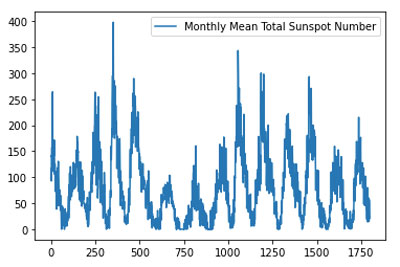## Engineering features

Currently, you only have one column (sunspots) as a feature, and it’s not possible to make predictions. In order to make the necessary predictions, you need the last sixty entries as input, and you’ll need to split that data into train and test sets:

``````# Convert the data values to numpy for better and faster processing
time_index = np.array(data['Date'])
data = np.array(data['Monthly Mean Total Sunspot Number'])

# ratio to split the data
SPLIT_RATIO = 0.8

# Dividing into train-test split
split_index = int(SPLIT_RATIO * data.shape)

# Train-Test Split
train_data = data[:split_index]
train_time = time_index[:split_index]
test_data = data[split_index:]
test_time = time_index[split_index:]``````

After creating the train and test sets, you need to create a function to prepare the input features. You don’t need to worry about calculating different things on the data for creating input features because TensorFlow does that for you. You just need to define the window size (how many entries you want as input features):

``````## required parameters
WINDOW_SIZE = 60
BATCH_SIZE = 32
SHUFFLE_BUFFER = 1000

## function to create the input features
def ts_data_generator(data, window_size, batch_size, shuffle_buffer):
'''
Utility function for time series data generation in batches
'''
ts_data = tf.data.Dataset.from_tensor_slices(data)
ts_data = ts_data.window(window_size + 1, shift=1, drop_remainder=True)
ts_data = ts_data.flat_map(lambda window: window.batch(window_size + 1))
ts_data = ts_data.shuffle(shuffle_buffer).map(lambda window: (window[:-1], window[-1]))
ts_data = ts_data.batch(batch_size).prefetch(1)
return ts_data# Expanding data into tensors

tensor_train_data = tf.expand_dims(train_data, axis=-1)
tensor_test_data = tf.expand_dims(test_data, axis=-1)

## generate input and output features for training and testing set
tensor_train_dataset = ts_data_generator(tensor_train_data, WINDOW_SIZE, BATCH_SIZE, SHUFFLE_BUFFER)
tensor_test_dataset = ts_data_generator(tensor_test_data, WINDOW_SIZE, BATCH_SIZE, SHUFFLE_BUFFER)``````

In the previous function, the data is converted into a TensorFlow dataset for faster processing. Then the window function is called to create different input features (the last sixty input entries as features). After that, the flat_map function is used to preserve the order of your time series by flattening the data. Finally, you shuffle and create batches of your data for training a DL model.

## Building a model and making predictions

The data is now ready for you to perform the time-series analysis on it. To make predictions, you need to create a DL-based model. In this case, you’ll be using a combination of CNN and long short-term memory (LSTM) models:

``````
## combination of 1D CNN and LSTM
input_shape=[None, 1]),
tf.keras.layers.LSTM(64, return_sequences=True),
tf.keras.layers.LSTM(64, return_sequences=True),
tf.keras.layers.Dense(30, activation="relu"),
tf.keras.layers.Dense(10, activation="relu"),
tf.keras.layers.Dense(1)])``````

In the previous code, a sequential model is initialized with a 1-D CNN layer, a few LSTM layers, and some Dense layers. The sequential class prepares a cascade pipeline of neural network layers in such a way that every layer that you define will be added to the previous layer. The input shape parameter is initialized with None, 1, indicating the shape of input that is provided to the model. In this case, a flattened input is provided, and the model’s shape is decided as 1.

Once you’ve defined the model, you need to compile it and train it on the training data:

``````## compile neural network model
optimizer = tf.keras.optimizers.SGD(lr=1e-3, momentum=0.9)
model.compile(loss=tf.keras.losses.Huber(),
optimizer=optimizer,
metrics=["mae"])
## training neural network model
history = model.fit(tensor_train_dataset, epochs=200, validation_data=tensor_test_dataset)``````

A few parameters are used to compile a model, including an optimizer algorithm that adjusts the weights of the network’s neurons and the learning rate, which reduces loss and improves overall accuracy. The loss of the procedure based on which weight modification occurs in the neural network is computed using a loss function. Then metrics are used to estimate the model’s overall accuracy. Meanwhile, mae is utilized because you want to forecast numerical data. Once the training starts, you should see your model running: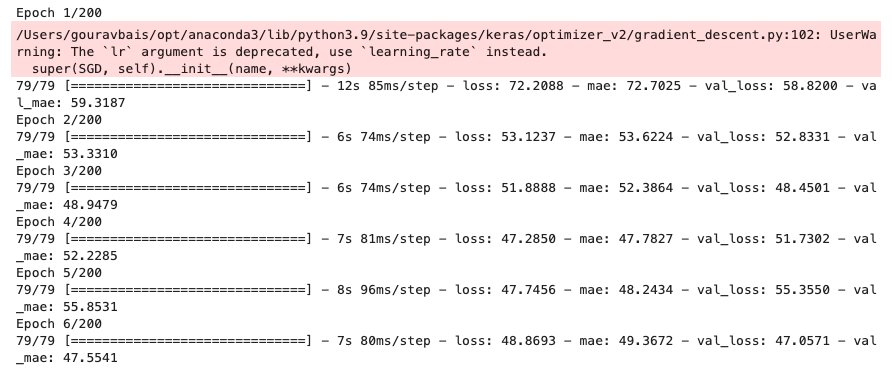The last line of the model is trained on training data with 200 epochs. You can check the training and validation losses of the model with the following code:

``````import matplotlib.pyplot as plt
# summarize history for loss
plt.plot(history.history['loss'])
plt.plot(history.history['val_loss'])
plt.title('model loss')
plt.ylabel('loss')
plt.xlabel('epoch')
plt.legend(['train', 'test'], loc='upper left')
plt.show()``````

Since a model is of no use if you can’t make predictions with it, you need to provide values for sixty days prior to the date you want to make a prediction for:

``````def model_forecast(model, data, window_size):
ds = tf.data.Dataset.from_tensor_slices(data)
ds = ds.window(window_size, shift=1, drop_remainder=True)
ds = ds.flat_map(lambda w: w.batch(window_size))
ds = ds.batch(32).prefetch(1)
forecast = model.predict(ds)
return forecast

rnn_forecast = model_forecast(model, data[..., np.newaxis], WINDOW_SIZE)
rnn_forecast = rnn_forecast[split_index - WINDOW_SIZE:-1, -1, 0]
# Overall Error
error = tf.keras.metrics.mean_absolute_error(test_data, rnn_forecast).numpy()
print(error)``````

Here, the model_forecast function is used, which is similar to ts_data_generator, except that it’s used for making predictions. The next two lines after the function are making the predictions for the test data. Meanwhile, the last line is used to show the error in predictions (the difference between actual test data and model predictions).

Now, your training and validation loss graph should look something like this:

PLACEHOLDER PLACEHOLDER

To check the accuracy of the predictions, you can plot the comparison graph using the following code:

``````import matplotlib.pyplot as plt
# summarize history for loss
plt.plot(test_data)
plt.plot(rnn_forecast)
plt.title('model loss')
plt.ylabel('loss')
plt.xlabel('epoch')
plt.legend(['test', 'predictions'], loc='upper left')
plt.show()``````

Your prediction graph will look something like this: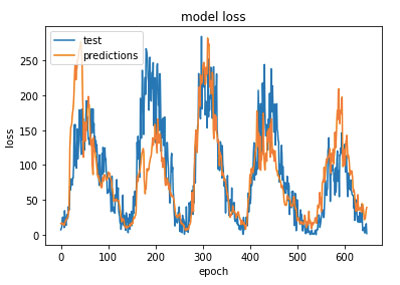As you can see, the predictions are good enough that they’re able to preserve the pattern of the time series, even though, at times, they are far from the actual result.

The entire code for this tutorial is available in this GitHub repo.

## Conclusion

In this article, you learned what time-series data is and how you can efficiently store it in time-series databases that are specifically designed for it, like InfluxDB.